# NCERT Solutions Class 10 Science Chapter 13 Magnetic Effects of Electric Current

Here you can get free NCERT Solutions for Class 10 Science, of Chapter 13 Magnetic Effects of Electric Current. All NCERT Book Solutions are given here exercise wise for Magnetic Effects of Electric Current. NCERT Solutions are helpful in the preparation of several school level, graduate and undergraduate level competitive exams. Practicing questions from NCERT Science Book, its Solutions for Class 10 Chapter 13 Magnetic Effects of Electric Current is proven to enhance your subject skills.

 Class: 10th Class Chapter: Chapter 13 Name: Magnetic Effects of Electric Current Subject: Science

## NCERT Solutions Class 10 Science Chapter 13 – Magnetic Effects of Electric Current

NCERT Class 10 Solutions Chapter 13 – Magnetic Effects of Electric Current are given below.

NCERT Solutions for Class 10 Science Chapter 13 Magnetic Effects of Electric Current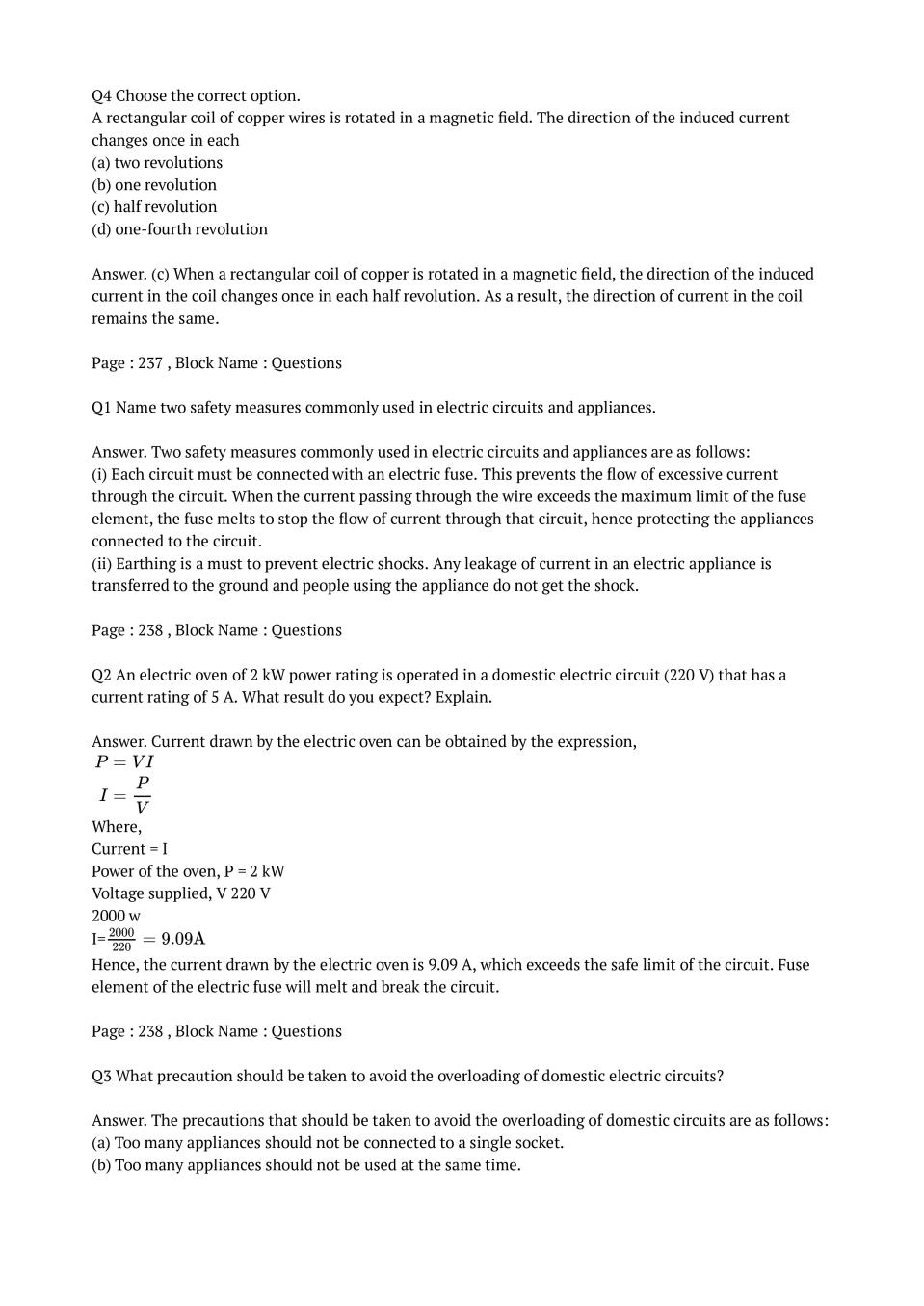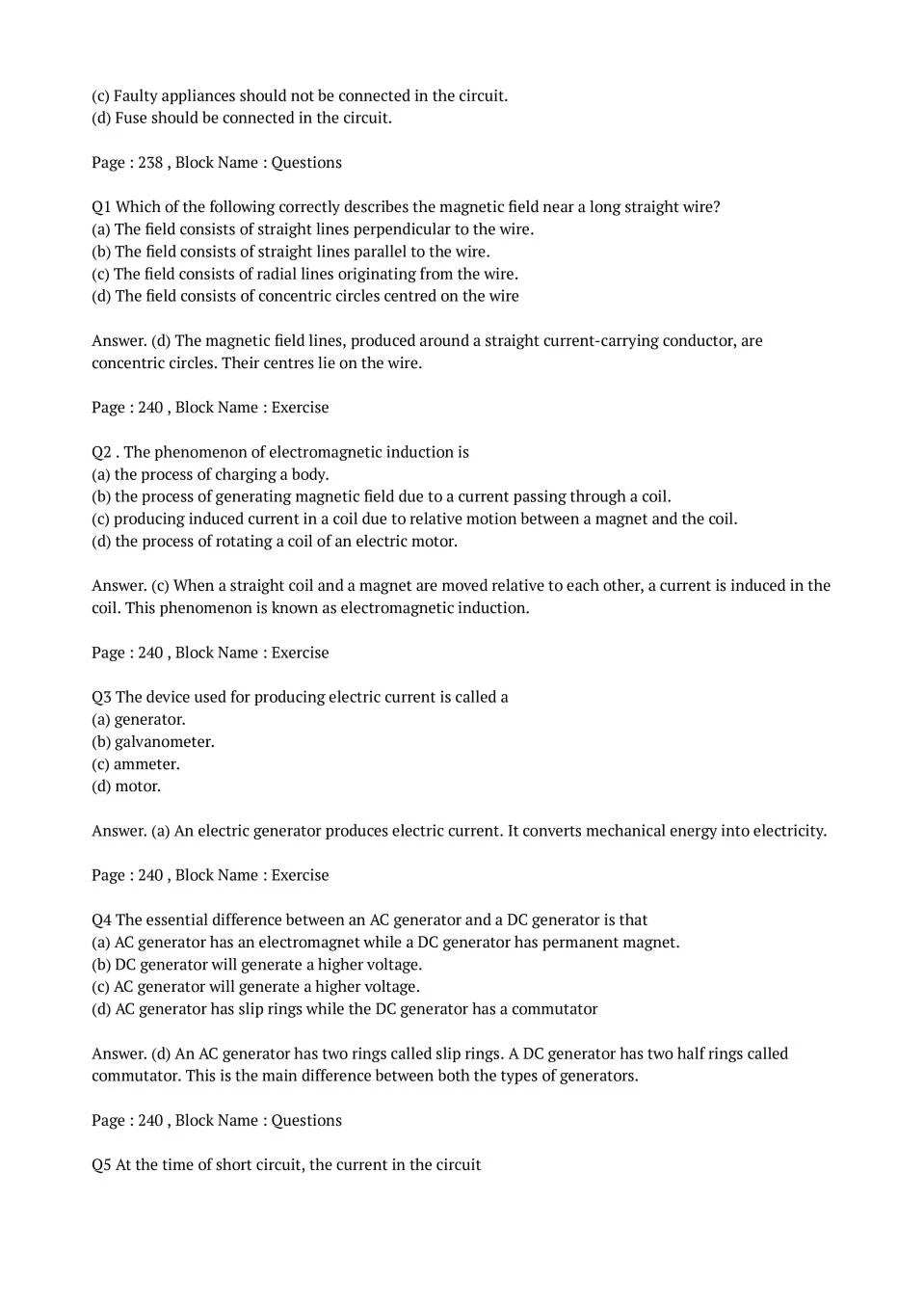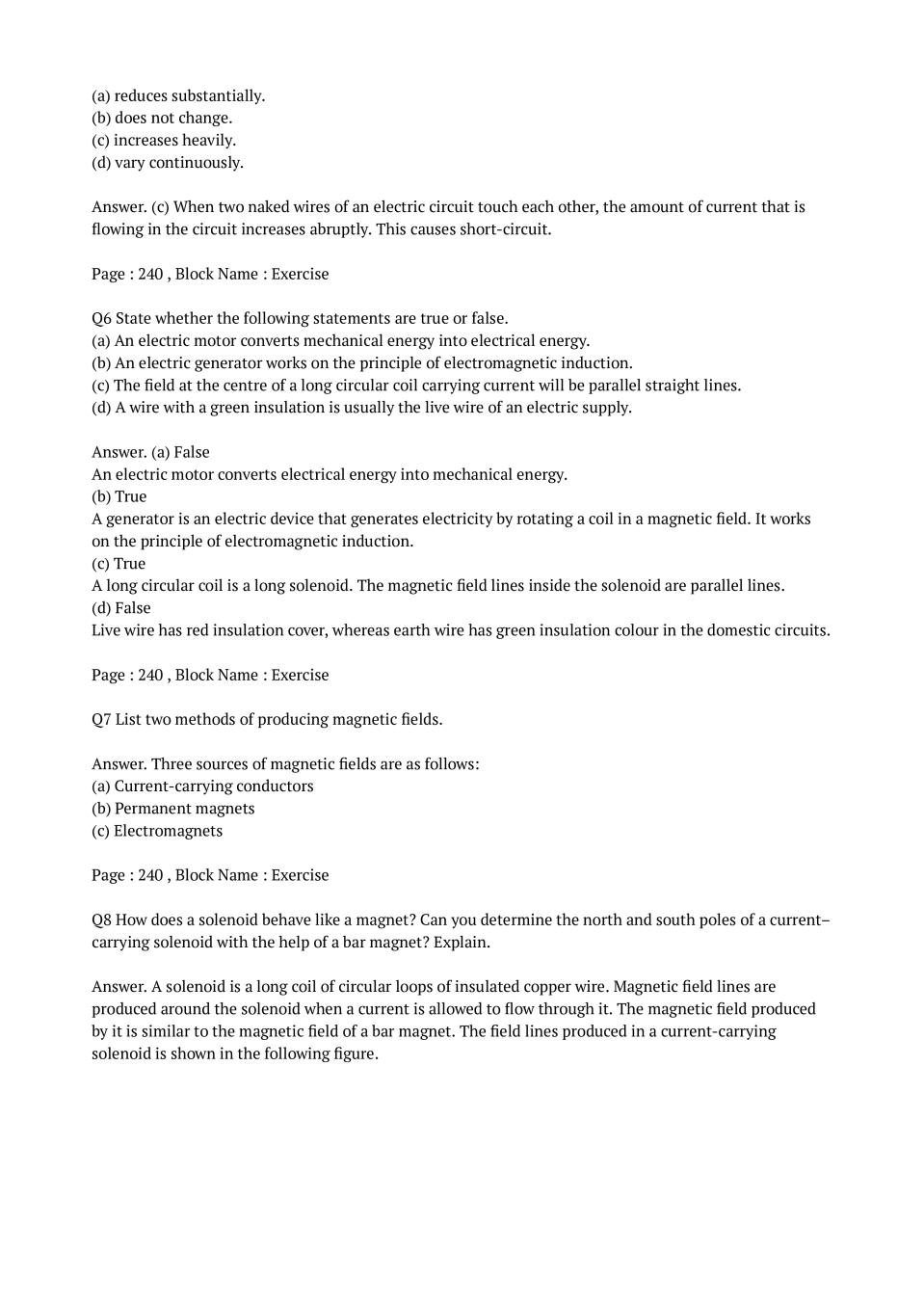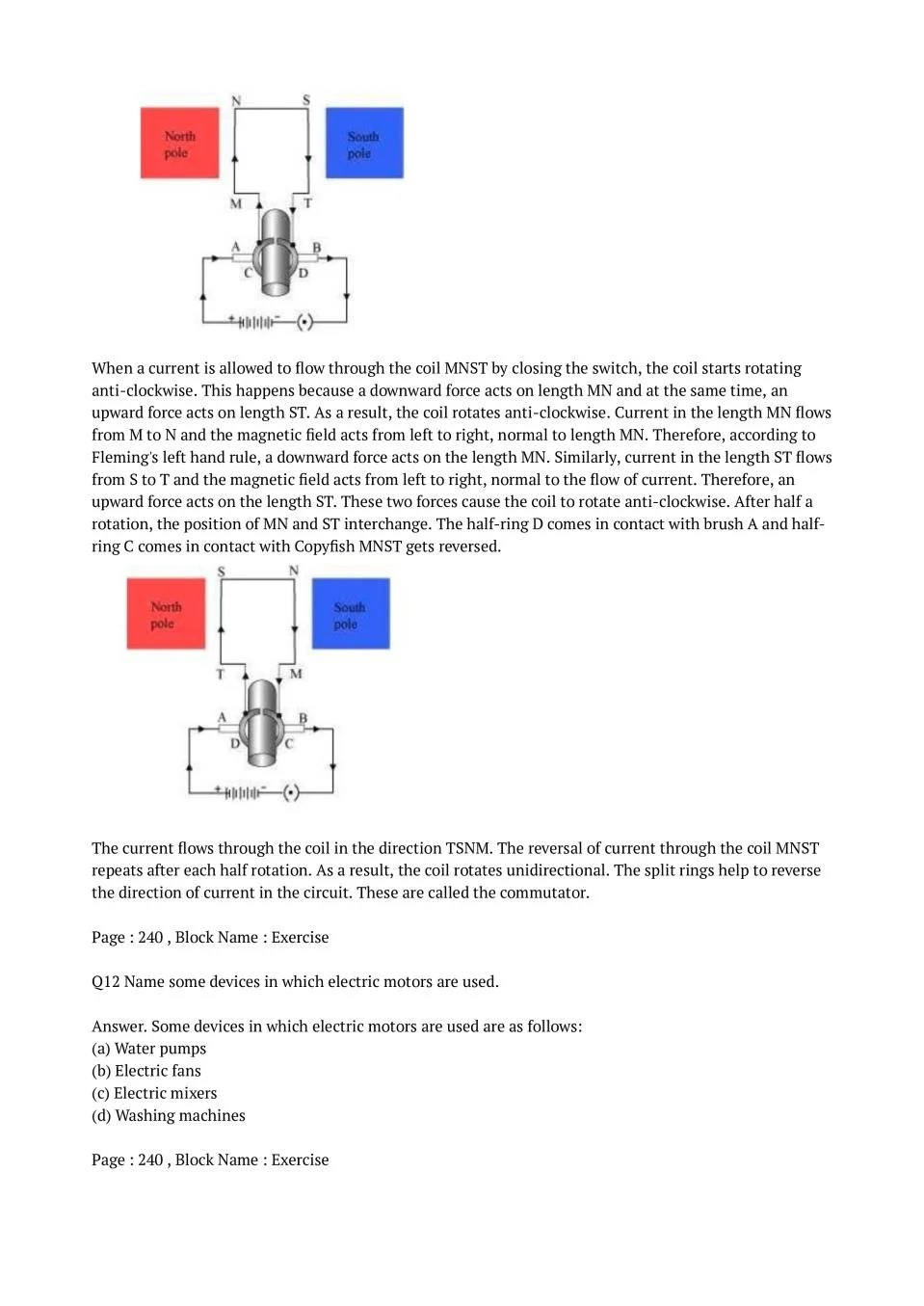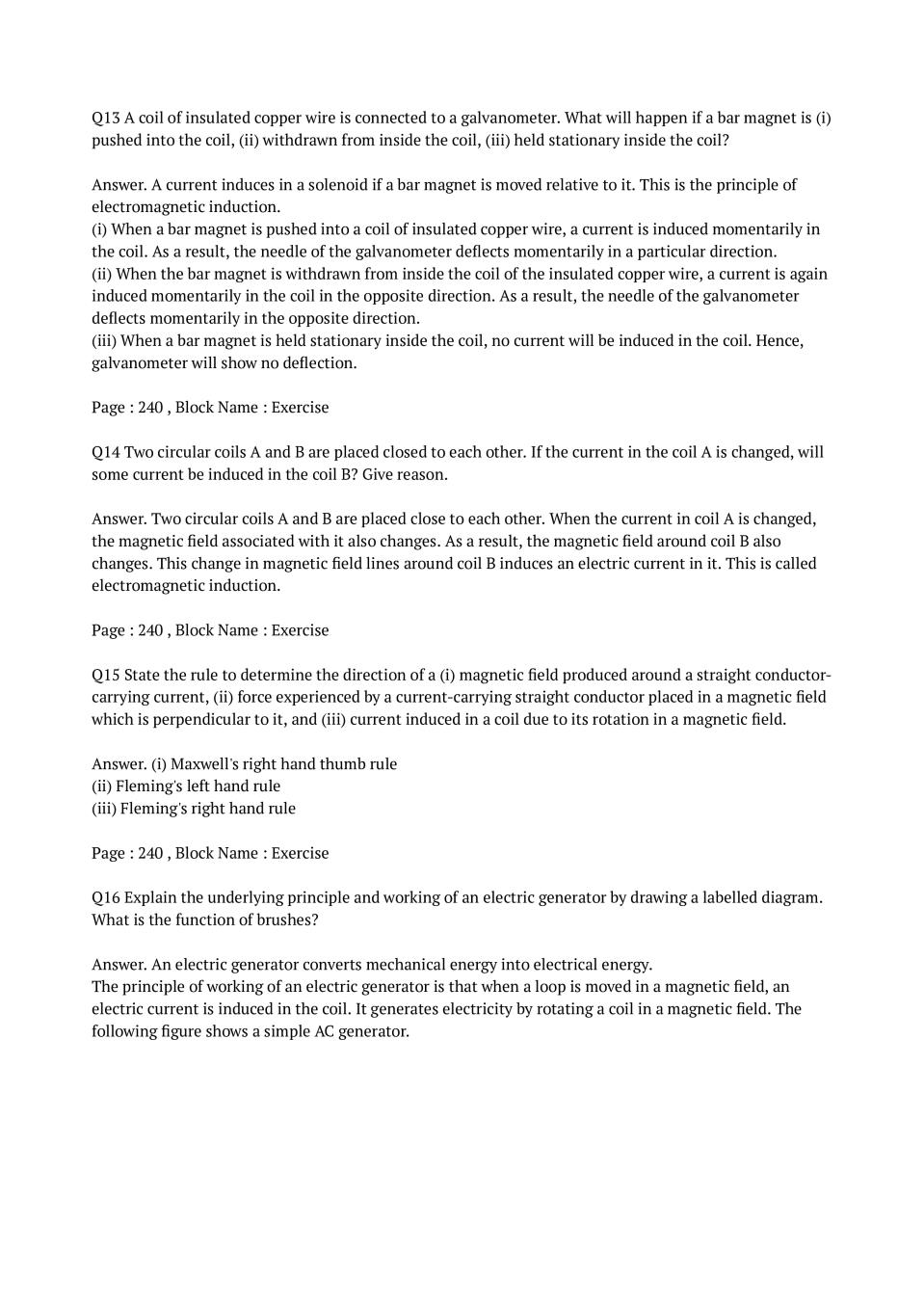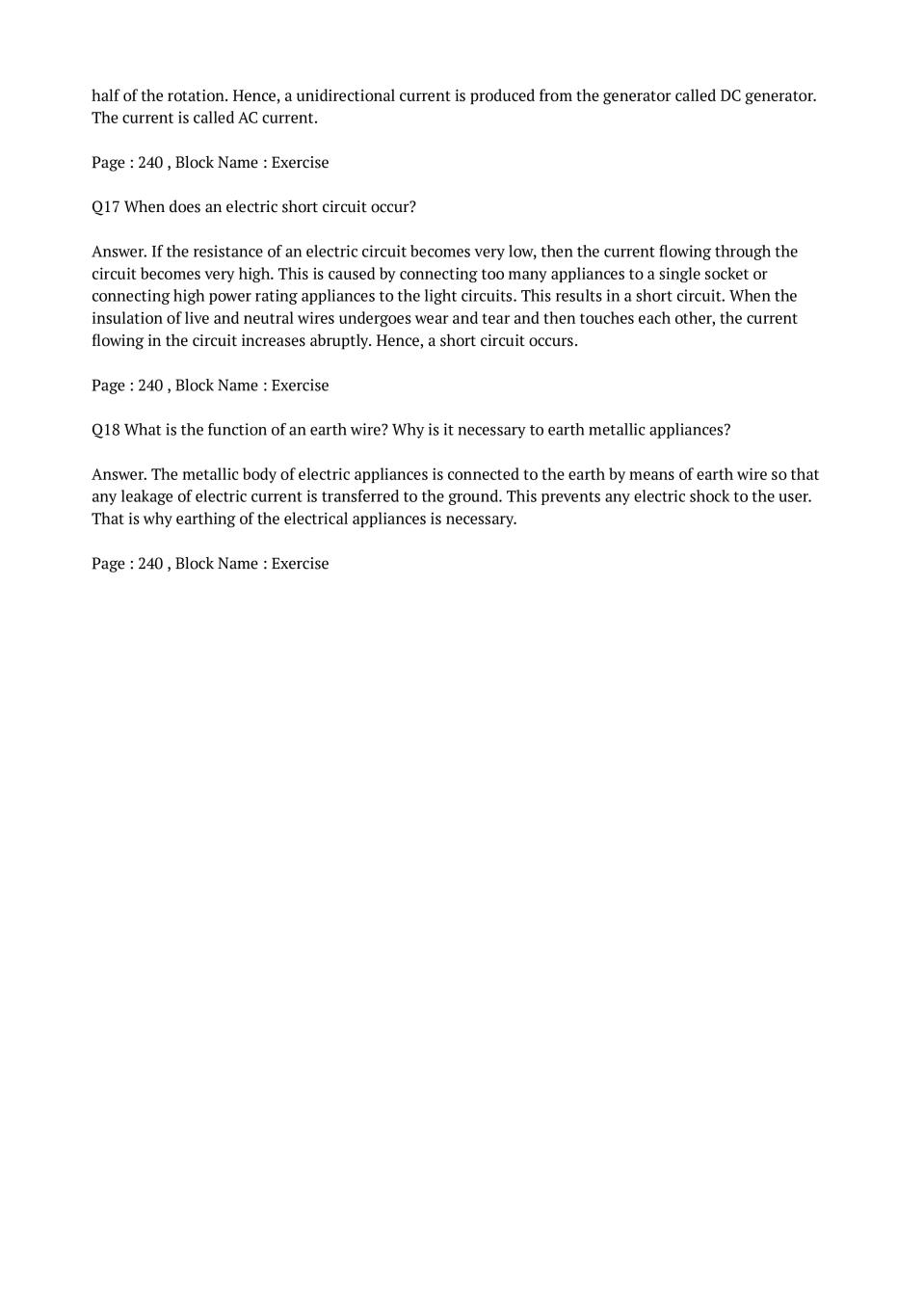### Class 10 Science Chapter 13 NCERT Solutions

Get step by step solutions for the questions given in class 10 Science textbook from the latest NCERT as per board exam guidelines.

### Class 10 Science Chapter 13 Important Topics

1. Magnetic Fields and Fields Lines
2. Magnetic Field due to a Current Carrying Conductor
–  Magnetic Field due to a Current through a Straight Conductor
–  Right-Hand Thumb Rule
–  Magnetic Field due to a Current through a Circular Loop
–  Magnetic Field due to a Current in a Solenoid
3. Force on A Current Carrying Conductor In a Magnetic Fields
4. Electric Motor
5. Electromagnetic Induction
6. Electric Generator
7. Domestic Electric Circuits

### Summary Chapter 13 Class 10 Science

• A compass needle is a small magnet. Its one end, which points towards north, is called a north pole, and the other end, which points towards south, is called a south pole.
• A magnetic field exists in the region surrounding a magnet, in which the force of the magnet can be detected.
• Field lines are used to represent a magnetic field. A field line is the path along which a hypothetical free north pole would tend to move. The direction of the magnetic field at a point is given by the direction that a north pole placed at that point would take. Field lines are shown closer together where the magnetic field is greater.
• A metallic wire carrying an electric current has associated with it a magnetic field. The field lines about the wire consist of a series of concentric circles whose direction is given by the right-hand rule.
• The pattern of the magnetic field around a conductor due to an electric current flowing through it depends on the shape of the conductor. The magnetic field of a solenoid carrying a current is similar to that of a bar magnet.
• An electromagnet consists of a core of soft iron wrapped around with a coil of insulated copper wire.
• A current-carrying conductor when placed in a magnetic field experiences a force. If the direction of the field and that of the current are mutually perpendicular to each other, then the force acting on the conductor will be perpendicular to both and will be given by Fleming’s left-hand rule. This is the basis of an electric motor. An electric motor is a device that converts electric energy into mechanical energy.
• The phenomenon of electromagnetic induction is the production of induced current in a coil placed in a region where the magnetic field changes with time. The magnetic field may change due to a relative motion between the coil and a magnet placed near to the coil. If the coil is placed near to a current-carrying conductor, the magnetic field may change either due to a change in the current through the conductor or due to the relative motion between the coil and conductor. The direction of the induced current is given by the Fleming’s right-hand rule.
• A generator converts mechanical energy into electrical energy. It works on the basis of electromagnetic induction.
• In our houses we receive AC electric power of 220 V with a frequency of 50 Hz. One of the wires in this supply is with red insulation, called live wire. The other one is of black insulation, which is a neutral wire. The potential difference between the two is 220 V. The third is the earth wire that has green insulation and this is connected to a metallic body deep inside earth. It is used as a safety measure to ensure that any leakage of current to a metallic body does not give any severe shock to a user.
• Fuse is the most important safety device, used for protecting the circuits due to short-circuiting or overloading of the circuits.

Exercise Covered under Science Book Class 10 Science Chapter 13 NCERT Solutions

Here on this page we have covered following exercises from NCERT Science Book for Class 10 Science Chapter 13 Magnetic Effects of Electric Current

 Questions All Questions Solved Exercises 18 Questions with Solutions

We also suggest you to practice all the solved examples given in the NCERT book to clear your concepts on Magnetic Effects of Electric Current. You can buy NCERT Books for Class 10 Science online for doorstep delivery.

### NCERT Solutions Class 10 Chapter Wise

If you want NCERT solutions of any topic other than Chapter 13 Magnetic Effects of Electric Current, check it from here. Science Book by NCERT has all chapters that are in your Class 10 syllabus.

### NCERT Solutions Class 10 All Subjects

All NCERT Solutions for Subjects other than Science are provided below:

 English Hindi Science Maths Social Science

### Other Classes NCERT Solutions

If you want NCERT solutions of any class other than Class 10, check it from here.